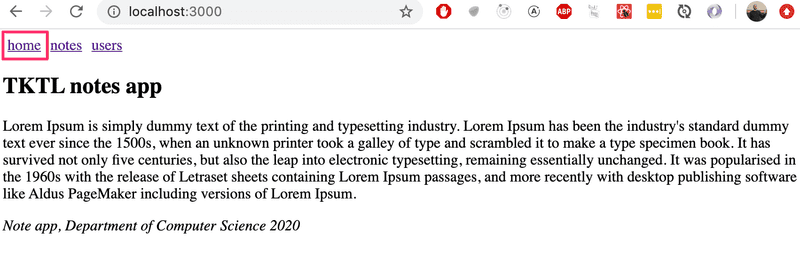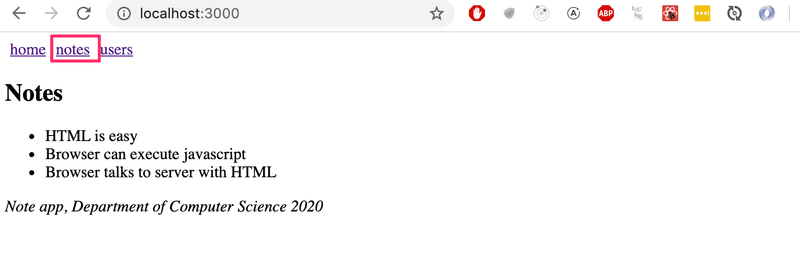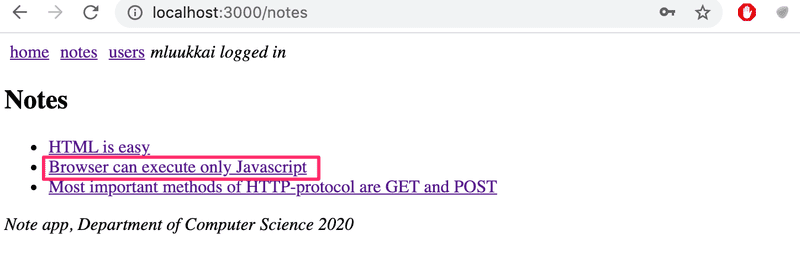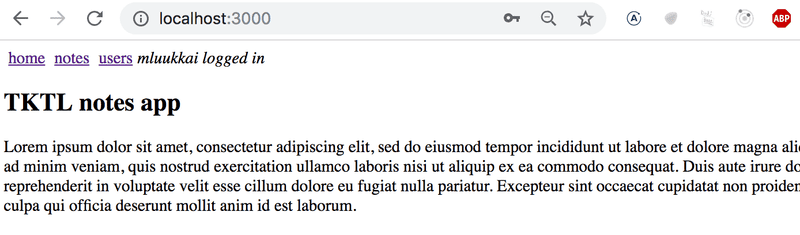a

# React-router``````import React, { useState }  from 'react'
import ReactDOM from 'react-dom/client'

const Home = () => (
<div> <h2>TKTL notes app</h2> </div>
)

const Notes = () => (
<div> <h2>Notes</h2> </div>
)

const Users = () => (
<div> <h2>Users</h2> </div>
)

const App = () => {
const [page, setPage] = useState('home')

const toPage = (page) => (event) => {
event.preventDefault()
setPage(page)
}

const content = () => {
if (page === 'home') {
return <Home />
} else if (page === 'notes') {
return <Notes />
} else if (page === 'users') {
return <Users />
}
}

}

return (
<div>
<div>
home
</a>
notes
</a>
users
</a>
</div>

{content()}
</div>
)
}

ReactDOM.createRoot(document.getElementById('root')).render(<App />, document.getElementById('root'))``````

### React Router

``npm install react-router-dom``

``````import {
BrowserRouter as Router,
} from "react-router-dom"

const App = () => {

}

return (
<Router>
<div>
</div>

<Routes>
<Route path="/notes" element={<Notes />} />
<Route path="/users" element={<Users />} />
<Route path="/" element={<Home />} />
</Routes>

<div>
<i>Note app, Department of Computer Science 2022</i>
</div>
</Router>
)
}``````

``````import {
BrowserRouter as Router,  Routes, Route, Link
} from "react-router-dom"``````

BrowserRouter是一个Router，使用HTML5历史API（pushState、replaceState和popState事件）来保持你的UI与URL同步。

``<Link to="/notes">notes</Link>``

``<Route path="/notes" element={<Notes />} />``

``````<Routes>
<Route path="/notes" element={<Notes />} />
<Route path="/users" element={<Users />} />
<Route path="/" element={<Home />} />
</Routes>``````

Routes的作用是渲染第一个路径与浏览器地址栏中的网址相匹配的组件。

### Parameterized route

Home and Users are unchanged from the previous exercise. Notes is a bit more complicated. It renders the list of notes passed to it as props in such a way that the name of each note is clickable.``````const Notes = ({notes}) => (
<div>
<h2>Notes</h2>
<ul>
{notes.map(note =>
<li key={note.id}>
</li>
)}
</ul>
</div>
)``````

``````<Router>
// ...

<Routes>
<Route path="/notes/:id" element={<Note notes={notes} />} />    <Route path="/notes" element={<Notes notes={notes} />} />
<Route path="/users" element={user ? <Users /> : <Navigate replace to="/login" />} />
<Route path="/" element={<Home />} />
</Routes>
</Router>``````

``<Route path="/notes/:id" element={<Note notes={notes} />} />``

``````import {
// ...
useParams} from "react-router-dom"

const Note = ({ notes }) => {
const id = useParams().id  const note = notes.find(n => n.id === Number(id))
return (
<div>
<h2>{note.content}</h2>
<div>{note.user}</div>
<div><strong>{note.important ? 'important' : ''}</strong></div>
</div>
)
}``````

Note组件接收所有的笔记作为propnotes，它可以通过React Router的useParams函数访问url参数（要显示的笔记的id）。

### useNavigate

``````<Router>
<div>

// ...
</Router>````````````import {
// ...
useNavigate} from 'react-router-dom'

const Login = (props) => {
const navigate = useNavigate()
const onSubmit = (event) => {
event.preventDefault()
navigate('/')  }

return (
<div>
<form onSubmit={onSubmit}>
<div>
</div>
<div>
</div>
</form>
</div>
)
}``````

useParamsuseNavigate都是钩子函数，就像我们现在已经多次使用的useState和useEffect。 正如你在第一章节所记得的，使用钩子函数有一些[规则](/en/part1/amorecomplexstatedebuggingreactapps/#rules-of-hooks）。Create-react-app已经被配置为在你违反这些规则时发出警告，例如，从条件语句中调用钩子函数。

### redirect

``<Route path="/users" element={user ? <Users /> : <Navigate replace to="/login" />} />``

``<Navigate replace to="/login" />``

``````const App = () => {
const [notes, setNotes] = useState([
// ...
])

const [user, setUser] = useState(null)

const login = (user) => {
setUser(user)
}

}

return (
<div>
<Router>
<div>
{user
? <em>{user} logged in</em>
}
</div>

<Routes>
<Route path="/notes/:id" element={<Note notes={notes} />} />
<Route path="/notes" element={<Notes notes={notes} />} />
<Route path="/users" element={user ? <Users /> : <Navigate replace to="/login" />} />
<Route path="/" element={<Home />} />
</Routes>
</Router>
<div>
<br />
<em>Note app, Department of Computer Science 2022</em>
</div>
</div>
)
}``````

### Parameterized route revisited

``````const Note = ({ notes }) => {
const id = useParams().id
const note = notes.find(n => n.id === Number(id))
// ...
}``````

``````const Note = ({ note }) => {
return (
<div>
<h2>{note.content}</h2>
<div>{note.user}</div>
<div><strong>{note.important ? 'important' : ''}</strong></div>
</div>
)
}``````

``````ReactDOM.createRoot(document.getElementById('root')).render(
<Router>    <App />
</Router>,  document.getElementById('root')
)``````

App组件变成。

``````import {
// ...
useMatch} from "react-router-dom"

const App = () => {
// ...

const match = useMatch('/notes/:id')  const note = match    ? notes.find(note => note.id === Number(match.params.id))    : null
return (
<div>
<div>
// ...
</div>

<Routes>
<Route path="/notes/:id" element={<Note note={note} />} />        <Route path="/notes" element={<Notes notes={notes} />} />
<Route path="/users" element={user ? <Users /> : <Navigate replace to="/login" />} />
<Route path="/" element={<Home />} />
</Routes>

<div>
<em>Note app, Department of Computer Science 2022</em>
</div>
</div>
)
}``````

``const match = useMatch('/notes/:id')``

``````const note = match
? notes.find(note => note.id === Number(match.params.id))
: null``````